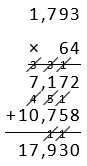# Multiplication and Division of Whole Numbers

## Objective

Multiply multi-digit numbers and assess the reasonableness of the product.

## Common Core Standards

### Core Standards

?

• 5.NBT.B.5 — Fluently multiply multi-digit whole numbers using the standard algorithm.

?

• 4.NBT.B.4

• 4.NBT.B.5

• 5.NBT.A.1

• 5.NBT.A.2

## Criteria for Success

?

1. Compute up to two-digit by four-digit and three-digit by three-digit products using the standard algorithm.
2. Predict whether estimated products will be greater than or less than the actual products, based on how the factors were rounded. (Optional)
3. Assess the reasonableness of an answer by comparing the actual product to the estimated product (MP.1).

#### Fishtank Plus

• Problem Set
• Student Handout Editor
• Vocabulary Package

?

### Problem 1

Use the digits 1 to 9, at most one time each, to create two numbers that have a product as close to 500,000 as possible.

You may use any length of factors as you would need, for example, eight-digit by one-digit or four-digit by three-digit.

#### References

Open Middle Multiplication of Large Numbers

Multiplication of Large Numbers by is made available on Open Middle under the CC BY-NC-SA 4.0 license. Accessed Dec. 7, 2018, 1:04 p.m..

Modified by The Match Foundation, Inc.

### Problem 2

Solve. Then estimate to assess the reasonableness of your answer. Then explain why your estimated product was greater or less than your actual product.

1.   1,882 x 296
2.   314 x 236
3.   4,920 x 408

#### References

EngageNY Mathematics Grade 5 Mathematics > Module 2 > Topic B > Lesson 8Concept Development

Grade 5 Mathematics > Module 2 > Topic B > Lesson 8 of the New York State Common Core Mathematics Curriculum from EngageNY and Great Minds. © 2015 Great Minds. Licensed by EngageNY of the New York State Education Department under the CC BY-NC-SA 3.0 US license. Accessed Dec. 2, 2016, 5:15 p.m..

Modified by The Match Foundation, Inc.

## Discussion of Problem Set

?

• What is the benefit of estimating before solving?
• Look at #1b and #1c. What do you notice about the estimated products? Analyze why the estimates are the same yet the products are so different. (You might point out the same issue in #1e and 1f.)
• How could the cost of the chairs have been found using mental math?
• In #3, Carmella estimated that she had 3,000 cards. How did she most likely round her factors?
• Would rounding the number of boxes of cards to 20 have been a better choice? Why or why not?
• Do we always have to round to a multiple of 10, 100, or 1,000? Is there a number between 10 and 20 that would have been a better choice for Carmella?
• Can you identify a situation in a real-life example where overestimating would be most appropriate?
• Can you identify a situation in the real world where underestimating would be most appropriate?

?

### Problem 1

For parts (a) and (b) below,

• Solve. Show or explain your work.
1. 910 x 233
2. 852 x 488

### Problem 2

This is Elmer’s work on a multiplication problem.1. Use estimation to explain why Elmer’s answer is not reasonable.
2. What error do you think Elmer made? Why do you think he made that error?
3. Find the correct product of 179 x 64.

#### References

Illustrative Mathematics Elmer's Multiplication Error

Elmer's Multiplication Error, accessed on Sept. 28, 2017, 3:18 p.m., is licensed by Illustrative Mathematics under either the CC BY 4.0 or CC BY-NC-SA 4.0. For further information, contact Illustrative Mathematics.

Modified by The Match Foundation, Inc.

?